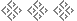Remembering Match Equities
Chris Bray, 1997## Match Equities

A match equity table gives you the percentage chance of winning a match at any particular score. Trailing 5–4 in a match to 7 points, your chances are 41 percent; leading 10–3 in a match to 15, they are 83 percent.

Three players — Robertie, Woolsey, and Kleinman — derived tables based on a mixture of mathematical theory and empirical evidence. Their three tables, however, had some differences. Over the years methods have become refined and the empirical evidence of real matches has greatly increased, so there is now general agreement on the table values.

The problem is, how do you remember the tables? A table for a 15-point match has 225 entries, a little too many to remember. Luckily help is at hand. I shall give two methods by which the match equity for any score in any length match can be calculated.

## Janowski Formula

The first, and most commonly used, is the Janowski formula, derived by Rick Janowski of Rochdale. If D is the difference between the two players’ scores and T is the number of points the trailing player needs to win, then the match-winning probability of the leading player is

50 +
 D × 85 T + 6
.

If the Crawford game is being played, this changes to

55 +
 D × 55 T + 2
.

This may seem daunting, but after a little practice it becomes quite easy to use.

## Underwood Formula

If you don’t like multiplication, then try the Underwood formula, named after the late U.S. player, Fleet Underwood. If T is the trailer’s score, L the leader’s score, and W the match length, then the leader’s match-winning probability is

50 + the first (LT) numbers from the following sequence:

 9 8 6 5 4 3 2 2 2 2 2 2

Then, if WL > 4, subtract

 (W − L) + (W− T) 4

from the number previously calculated.

Again, this may seem complex but it is surprisingly easy to use. For example, leading 5–2 in a match to 11, the leader’s probability of winning is

50 + 9 + 8 + 6 −
 6 + 9 4
,

which is 69 percent.

Of the two formulas, the Janowski is slightly more accurate, particularly for longer matches where one player has a significant lead.